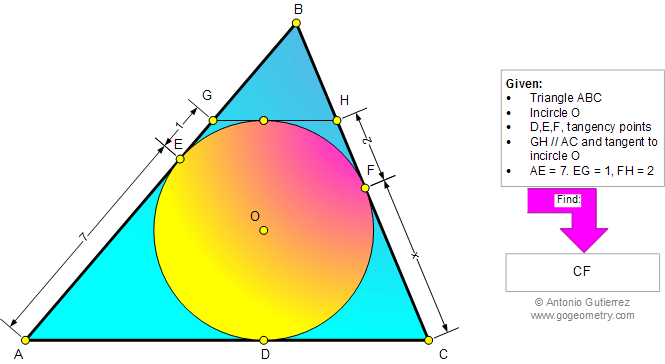###### Online Geometry Problem 861: Triangle, Incircle, Parallel, Tangent, Metric Relations. Level: High School, College, Mathematics Education
 The figure below shows a triangle ABC with the incircle O tangent to AB, BC, and AC at E, F, and D, respectively. GH is parallel to AC and tangent to the circle O.If AE = 7, EG = 1, and FH = 2, find CF.Home | Search | Geometry | Problems | All Problems | Open Problems | Visual Index | 10 Problems | 861-870 | Triangles | Incircle | Circles | Circle Tangent Line | Parallel | Email | Solution / comment | By Antonio Gutierrez